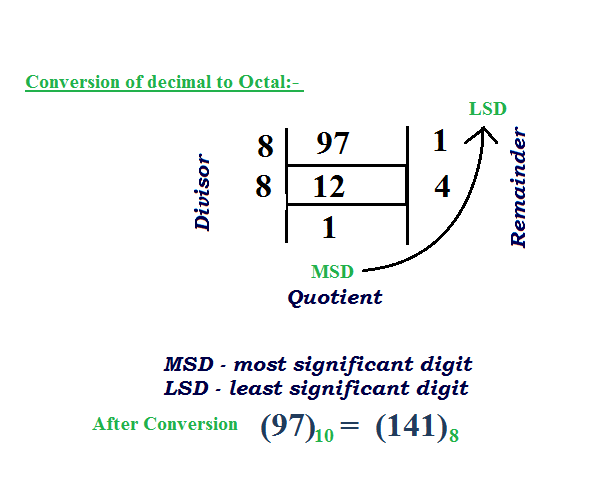Related Articles

# Check if number is palindrome or not in Octal

• Difficulty Level : Easy
• Last Updated : 23 Feb, 2021

Given a number which may be in octal or in decimal. If the number is not octal then convert it into octal then check if it is palindrome or not. If it is palindrome then print 1 otherwise print 0. If the number is already in octal then check if the number is palindrome or not.
Examples :

```Input :n = 111
Output : Yes
Explanation:
all digits of 111 are in 0-7 so it
is a octal number. Read 111 the result
is same backward and forward so it
is a palindrome number.

Input : 68
Output : No
Explanation:
68 is not a octal number because it's
all digits are not in 0-7. So first we
need to convert it into octal
(68)base10(Decimal) = (104)base8(octal)
104 is not palindrome.

Input : 97
Output : Yes
Explanation:
97 is not a octal number because it's all
digits are not in 0-7 so first we need to
convert it into decimal to octal
(97)base10(Decimal) = (141)base8(octal)
141 is palindrome so output = 1.```

Octal Number : The octal numeral system, or oct for short, is the base-8 number system, and uses the digits 0 to 7.
How to Convert Decimal Number to Octal Number :## C++

 `// C++ program to check if octal``// representation of a number is prime``#include ``using` `namespace` `std;` `const` `int` `MAX_DIGITS = 20;` `/* Function to Check no is in octal``or not */``bool` `isOctal(``long` `n)``{``    ``while` `(n)``    ``{``        ``if` `((n % 10) >= 8)``            ``return` `false``;``        ``else``            ``n = n / 10;``    ``}``    ``return` `true``;``}` `/* Function To check no is palindrome``or not*/``int` `isPalindrome(``long` `n)``{``    ``// If number is already in octal, we traverse``    ``// digits using repeated division with 10. Else``    ``// we traverse digits using repeated division``    ``// with 8``    ``int` `divide = (isOctal(n) == ``false``)? 8 : 10;` `    ``// To store individual digits``    ``int` `octal[MAX_DIGITS];` `    ``// Traversing all digits``    ``int` `i = 0;``    ``while` `(n != 0)``    ``{``        ``octal[i++] = n % divide;``        ``n = n / divide;``    ``}` `    ``// checking if octal no is palindrome``    ``for` `(``int` `j = i - 1, k = 0; k <= j; j--, k++)``        ``if` `(octal[j] != octal[k])``            ``return` `false``;` `    ``return` `true``;``}` `// Driver code``int` `main()``{``    ``long` `n = 97;``    ``if` `(isPalindrome(n))``    ``cout << ``"Yes"``;``    ``else``    ``cout << ``"No"``;``    ``return` `0;``}`

## Java

 `// Java program to check if``// octal representation of``// a number is prime``import` `java.io.*;``import` `java.util.*;``import` `java.lang.*;` `class` `GFG``{``static` `int` `MAX_DIGITS = ``20``;` `/* Function to Check no``is in octal or not */``static` `int` `isOctal(``int` `n)``{``    ``while` `(n > ``0``)``    ``{``        ``if` `((n % ``10``) >= ``8``)``            ``return` `0``;``        ``else``            ``n = n / ``10``;``    ``}``    ``return` `1``;``}` `/* Function To check no``is palindrome or not*/``static` `int` `isPalindrome(``int` `n)``{``    ``// If number is already in``    ``// octal, we traverse digits``    ``// using repeated division``    ``// with 10. Else we traverse``    ``// digits using repeated``    ``// division with 8``    ``int` `divide = (isOctal(n) == ``0``) ? ``8` `: ``10``;` `    ``// To store individual digits``    ``int` `octal[] = ``new` `int``[MAX_DIGITS];` `    ``// Traversing all digits``    ``int` `i = ``0``;``    ``while` `(n != ``0``)``    ``{``        ``octal[i++] = n % divide;``        ``n = n / divide;``    ``}` `    ``// checking if octal``    ``// no is palindrome``    ``for` `(``int` `j = i - ``1``,``            ``k = ``0``; k <= j; j--, k++)``        ``if` `(octal[j] != octal[k])``            ``return` `0``;` `    ``return` `1``;``}` `// Driver Code``public` `static` `void` `main(String[] args)``{``    ``int` `n = ``97``;``    ``if` `(isPalindrome(n) > ``0``)``    ``System.out.print(``"Yes"``);``    ``else``    ``System.out.print(``"No"``);``}``}` `// This code is contributed``// by Akanksha Rai(Abby_akku)`

## Python3

 `# Python3 program to check if``# octal representation of``# a number is prime``MAX_DIGITS ``=` `20``;` `# Function to Check no``# is in octal or not``def` `isOctal(n):``    ``while``(n):``        ``if``((n ``%` `10``) >``=` `8``):``            ``return` `False``        ``else``:``            ``n ``=` `int``(n ``/` `10``)``    ``return` `True` `# Function To check no``# is palindrome or not``def` `isPalindrome(n):``    ``# If number is already in``    ``# octal, we traverse digits``    ``# using repeated division``    ``# with 10. Else we traverse``    ``# digits using repeated``    ``# division with 8``    ``divide ``=` `8` `if``(isOctal(n) ``=``=` `False``) ``else` `10` `    ``# To store individual digits``    ``octal``=``[]` `    ``# Traversing all digits``    ``while` `(n !``=` `0``):``        ``octal.append(n ``%` `divide)``        ``n ``=` `int``(n ``/` `divide)` `    ``# checking if octal``    ``# no is palindrome``    ``j ``=` `len``(octal)``-``1``    ``k ``=` `0``    ``while``(k <``=` `j):``        ``if``(octal[j] !``=` `octal[k]):``            ``return` `False``        ``j``-``=``1``        ``k``+``=``1``    ``return` `True`  `# Driver Code``if` `__name__``=``=``'__main__'``:``    ``n ``=` `97``;``    ``if` `(isPalindrome(n)):``        ``print``(``"Yes"``)``    ``else``:``        ``print``(``"No"``)` `# This code is contributed by mits`

## C#

 `// C# program to check if``// octal representation of``// a number is prime``using` `System;` `class` `GFG``{``static` `long` `MAX_DIGITS = 20;` `/* Function to Check no``is in octal or not */``static` `long` `isOctal(``long` `n)``{``    ``while` `(n > 0)``    ``{``        ``if` `((n % 10) >= 8)``            ``return` `0;``        ``else``            ``n = n / 10;``    ``}``    ``return` `1;``}` `/* Function To check no``is palindrome or not*/``static` `long` `isPalindrome(``long` `n)``{``    ``// If number is already in``    ``// octal, we traverse digits``    ``// using repeated division``    ``// with 10. Else we traverse``    ``// digits using repeated``    ``// division with 8``    ``long` `divide = (isOctal(n) == 0) ? 8 : 10;` `    ``// To store individual digits``    ``long``[] octal = ``new` `long``[MAX_DIGITS];` `    ``// Traversing all digits``    ``long` `i = 0;``    ``while` `(n != 0)``    ``{``        ``octal[i++] = n % divide;``        ``n = n / divide;``    ``}` `    ``// checking if octal``    ``// no is palindrome``    ``for` `(``long` `j = i - 1,``            ``k = 0; k <= j; j--, k++)``        ``if` `(octal[j] != octal[k])``            ``return` `0;` `    ``return` `1;``}` `// Driver Code``static` `int` `Main()``{``    ``long` `n = 97;``    ``if` `(isPalindrome(n) > 0)``    ``Console.Write(``"Yes"``);``    ``else``    ``Console.Write(``"No"``);``    ``return` `0;``}``}` `// This code is contributed``// by mits`

## PHP

 `= 8)``            ``return` `false;``        ``else``            ``\$n` `= (int)``\$n` `/ 10;``    ``}``    ``return` `true;``}` `// Function To check no``// is palindrome or not``function` `isPalindrome(``\$n``)``{``    ``global` `\$MAX_DIGITS``;``    ``// If number is already in``    ``// octal, we traverse digits``    ``// using repeated division``    ``// with 10. Else we traverse``    ``// digits using repeated``    ``// division with 8``    ``\$divide` `= (isOctal(``\$n``) ==``                ``false) ? 8 : 10;` `    ``// To store individual digits``    ``\$octal``;` `    ``// Traversing all digits``    ``\$i` `= 0;``    ``while` `(``\$n` `!= 0)``    ``{``        ``\$octal``[``\$i``++] = ``\$n` `% ``\$divide``;``        ``\$n` `= (int)``\$n` `/ ``\$divide``;``    ``}` `    ``// checking if octal``    ``// no is palindrome``    ``for` `(``\$j` `= ``\$i` `- 1, ``\$k` `= 0;``        ``\$k` `<= ``\$j``; ``\$j``--, ``\$k``++)``        ``if` `(``\$octal``[``\$j``] != ``\$octal``[``\$k``])``            ``return` `-1;` `    ``return` `0;``}` `// Driver Code``\$n` `= 97;``if` `(isPalindrome(``\$n``))``echo` `"Yes"``;``else``echo` `"No"``;` `// This code is contributed by ajit``?>`

## Javascript

 ``

Output :

`Yes`

This article is contributed by R_Raj. If you like GeeksforGeeks and would like to contribute, you can also write an article using contribute.geeksforgeeks.org or mail your article to contribute@geeksforgeeks.org. See your article appearing on the GeeksforGeeks main page and help other Geeks.